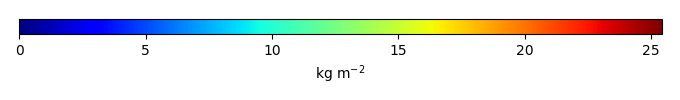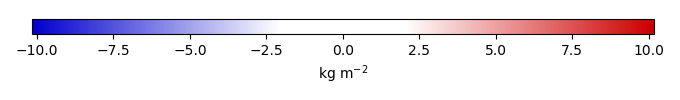# Mean State

Period Mean (original grids) [Pg]
Model Period Mean (intersection) [Pg]
Model Period Mean (complement) [Pg]
Benchmark Period Mean (intersection) [Pg]
Benchmark Period Mean (complement) [Pg]
Bias [kg m-2]
Bias Score 
Spatial Distribution Score 
Overall Score 
Benchmark [-] 38.7
CLM5PHSOFF [-] 47.1 46.4 0.867 38.7 0.0457 1.70 0.67 0.85 0.76
CLM5PHSON [-] 38.5 38.7 0.202 38.7 0.0457 0.293 0.77 0.95 0.86
Period Mean (original grids) [Pg]
Model Period Mean (intersection) [Pg]
Model Period Mean (complement) [Pg]
Benchmark Period Mean (intersection) [Pg]
Benchmark Period Mean (complement) [Pg]
Bias [kg m-2]
Bias Score 
Spatial Distribution Score 
Overall Score 
Benchmark [-] 11.9
CLM5PHSOFF [-] 2.31 1.97 0.0401 11.9 0.0196 -1.49 0.42 0.51 0.47
CLM5PHSON [-] 3.25 2.85 0.0430 11.9 0.0196 -1.35 0.45 0.59 0.52
Period Mean (original grids) [Pg]
Model Period Mean (intersection) [Pg]
Model Period Mean (complement) [Pg]
Benchmark Period Mean (intersection) [Pg]
Benchmark Period Mean (complement) [Pg]
Bias [kg m-2]
Bias Score 
Spatial Distribution Score 
Overall Score 
Benchmark [-] 24.6
CLM5PHSOFF [-] 10.4 8.53 1.41 24.5 0.0612 -1.72 0.44 0.72 0.58
CLM5PHSON [-] 12.3 11.7 0.367 24.5 0.0612 -1.21 0.51 0.80 0.65
Period Mean (original grids) [Pg]
Model Period Mean (intersection) [Pg]
Model Period Mean (complement) [Pg]
Benchmark Period Mean (intersection) [Pg]
Benchmark Period Mean (complement) [Pg]
Bias [kg m-2]
Bias Score 
Spatial Distribution Score 
Overall Score 
Benchmark [-] 36.8
CLM5PHSOFF [-] 78.2 66.6 9.40 35.7 1.10 8.86 0.61 0.92 0.76
CLM5PHSON [-] 39.9 37.9 1.80 35.7 1.10 5.14 0.72 0.92 0.82
Period Mean (original grids) [Pg]
Model Period Mean (intersection) [Pg]
Model Period Mean (complement) [Pg]
Benchmark Period Mean (intersection) [Pg]
Benchmark Period Mean (complement) [Pg]
Bias [kg m-2]
Bias Score 
Spatial Distribution Score 
Overall Score 
Benchmark [-] 38.4
CLM5PHSOFF [-] 27.7 22.1 5.53 38.3 0.0891 -2.14 0.55 0.81 0.68
CLM5PHSON [-] 31.6 23.2 9.15 38.3 0.0891 -1.53 0.59 0.80 0.69
Period Mean (original grids) [Pg]
Model Period Mean (intersection) [Pg]
Model Period Mean (complement) [Pg]
Benchmark Period Mean (intersection) [Pg]
Benchmark Period Mean (complement) [Pg]
Bias [kg m-2]
Bias Score 
Spatial Distribution Score 
Overall Score 
Benchmark [-] 43.7
CLM5PHSOFF [-] 32.8 27.7 3.21 43.4 0.257 -2.24 0.56 0.82 0.69
CLM5PHSON [-] 24.8 23.2 0.670 43.4 0.257 -1.98 0.61 0.89 0.75
Period Mean (original grids) [Pg]
Model Period Mean (intersection) [Pg]
Model Period Mean (complement) [Pg]
Benchmark Period Mean (intersection) [Pg]
Benchmark Period Mean (complement) [Pg]
Bias [kg m-2]
Bias Score 
Spatial Distribution Score 
Overall Score 
Benchmark [-] 13.7
CLM5PHSOFF [-] 18.2 3.44 14.2 13.5 0.139 -1.61 0.41 0.60 0.50
CLM5PHSON [-] 11.0 3.66 7.16 13.5 0.139 -1.38 0.44 0.64 0.54
Period Mean (original grids) [Pg]
Model Period Mean (intersection) [Pg]
Model Period Mean (complement) [Pg]
Benchmark Period Mean (intersection) [Pg]
Benchmark Period Mean (complement) [Pg]
Bias [kg m-2]
Bias Score 
Spatial Distribution Score 
Overall Score 
Benchmark [-] 352.
CLM5PHSOFF [-] 542. 370. 172. 348. 3.81 0.288 0.56 0.74 0.65
CLM5PHSON [-] 486. 309. 178. 348. 3.81 0.0653 0.64 0.85 0.75
Period Mean (original grids) [Pg]
Model Period Mean (intersection) [Pg]
Model Period Mean (complement) [Pg]
Benchmark Period Mean (intersection) [Pg]
Benchmark Period Mean (complement) [Pg]
Bias [kg m-2]
Bias Score 
Spatial Distribution Score 
Overall Score 
Benchmark [-] 2.53
CLM5PHSOFF [-] 0.976 0.0715 0.806 2.53 0.00250 -1.11 0.38 0.0030 0.19
CLM5PHSON [-] 1.55 0.211 1.35 2.53 0.00250 -1.04 0.39 0.021 0.21
Period Mean (original grids) [Pg]
Model Period Mean (intersection) [Pg]
Model Period Mean (complement) [Pg]
Benchmark Period Mean (intersection) [Pg]
Benchmark Period Mean (complement) [Pg]
Bias [kg m-2]
Bias Score 
Spatial Distribution Score 
Overall Score 
Benchmark [-] 11.1
CLM5PHSOFF [-] 16.3 13.5 1.99 10.9 0.149 0.792 0.54 0.67 0.60
CLM5PHSON [-] 10.2 9.38 0.547 10.9 0.149 0.813 0.61 0.77 0.69
Period Mean (original grids) [Pg]
Model Period Mean (intersection) [Pg]
Model Period Mean (complement) [Pg]
Benchmark Period Mean (intersection) [Pg]
Benchmark Period Mean (complement) [Pg]
Bias [kg m-2]
Bias Score 
Spatial Distribution Score 
Overall Score 
Benchmark [-] 1.55
CLM5PHSOFF [-] 7.10 1.11 5.64 1.52 0.0247 -0.473 0.50 0.72 0.61
CLM5PHSON [-] 11.9 1.32 10.5 1.52 0.0247 0.434 0.55 0.68 0.62
Period Mean (original grids) [Pg]
Model Period Mean (intersection) [Pg]
Model Period Mean (complement) [Pg]
Benchmark Period Mean (intersection) [Pg]
Benchmark Period Mean (complement) [Pg]
Bias [kg m-2]
Bias Score 
Spatial Distribution Score 
Overall Score 
Benchmark [-] 0.219
CLM5PHSOFF [-] 1.45 0.0491 1.50 0.219 0.00 -0.542 0.42 0.020 0.22
CLM5PHSON [-] 3.04 0.0844 2.99 0.219 0.00 -0.433 0.47 0.082 0.27
Period Mean (original grids) [Pg]
Model Period Mean (intersection) [Pg]
Model Period Mean (complement) [Pg]
Benchmark Period Mean (intersection) [Pg]
Benchmark Period Mean (complement) [Pg]
Bias [kg m-2]
Bias Score 
Spatial Distribution Score 
Overall Score 
Benchmark [-] 100.
CLM5PHSOFF [-] 149. 147. 1.94 100. 0.0690 5.46 0.59 0.72 0.65
CLM5PHSON [-] 128. 128. 0.396 100. 0.0690 3.63 0.71 0.92 0.81
Period Mean (original grids) [Pg]
Model Period Mean (intersection) [Pg]
Model Period Mean (complement) [Pg]
Benchmark Period Mean (intersection) [Pg]
Benchmark Period Mean (complement) [Pg]
Bias [kg m-2]
Bias Score 
Spatial Distribution Score 
Overall Score 
Benchmark [-] 18.4
CLM5PHSOFF [-] 20.6 19.4 1.25 18.4 0.0281 0.100 0.50 0.52 0.51
CLM5PHSON [-] 21.8 21.8 0.347 18.4 0.0281 0.735 0.55 0.58 0.56
Period Mean (original grids) [Pg]
Model Period Mean (intersection) [Pg]
Model Period Mean (complement) [Pg]
Benchmark Period Mean (intersection) [Pg]
Benchmark Period Mean (complement) [Pg]
Bias [kg m-2]
Bias Score 
Spatial Distribution Score 
Overall Score 
Benchmark [-] 7.28
CLM5PHSOFF [-] 8.11 7.83 0.482 7.15 0.128 0.290 0.48 0.50 0.49
CLM5PHSON [-] 6.25 6.14 0.145 7.15 0.128 0.387 0.52 0.57 0.55

# Temporally integrated period mean

BENCHMARK MEANMODEL MEANBIASBIAS SCORESPATIAL TAYLOR DIAGRAMMODEL COLORS# Spatially integrated regional mean

MODEL COLORS# All Models

BenchmarkCLM5PHSOFFCLM5PHSON# Data Information

title: Global Tropical Forest Biomass

institution: NASA-JPL (NASA Jet Propulsion Laboratory, California Institute of Technology, Pasadena, California 91109, USA)

institute_id: NASA-JPL

source: 4079 in situ inventory plots, satellite light detection and ranging (LiDAR) samples of forest structure, and optical and microwave imagery (1-km resolution) to extrapolate estimates

history: 2015-01-08-T06:37:41Z Converted from 1 km x 1 km to 0.5 degree x 0.5 degree by Mingquan Mu (mmu@uci.edu) on Fri Jan 9 10:37:41 PST 2015

creation_date: 2015-01-08-T06:37:41Z

Conventions: CF-1.6

product: observations

data_structure: grid

realm: land

contact: Prof. James T. Randerson (jranders@uci.edu) or Dr. Mingquan Mu (mmu@uci.edu)

references: Saatchi, S. S., N. L. Harris, S. Brown, M. Lefsky, E. T. A. Mitchard, W. Salas, B. R. Zutta, W. Buermann, S. L. Lewis, S. Hagen, S. Petrova, L. White, M. Silman, and A. Morel (2011), Benchmark map of forest carbon stocks in tropical regions across three continents, Proc. Nat. Acad. Sci., 108(24):9899-9904, doi:10.1073/pnas.1019576108.